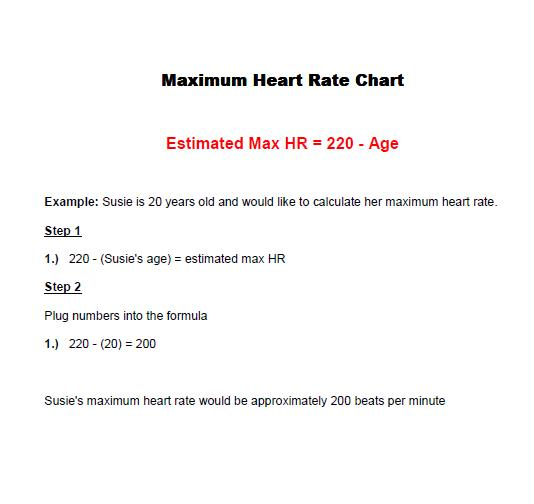# Calculate Your Maximum Heart Rate

Your maximum heart rate is the number of times your heart can beat in a minute. The most popular method for determining maximum heart rate is to subtract your age from 220 if you are a man. For women, subtract your age from 226. Keep in mind this method is only a quick estimate of your max heart rate.

Note: Your maximum heart rate is only used to help you determine your target heart rate. Below is a simple way to calculate your estimated maximum heart rate.

Maximum heart rate calculator

# MAXIMUM HEART RATE CALCULATOR

Years *Your entered year is out of our calculation *Please enter the years

Your estimated maximum heart rate is : 120 bpm

Maximum Heart Rate Formula

1.) 220 - age = estimated max HR

Example: Susie is 20 years old and would like to calculate her maximum heart rate.

Step 1

1.)   220 - (Susie's age) = estimated max HR

Step 2

Plug numbers into the formula

1.)    220 - (20) = 200

Susie's maximum heart rate would be approximately 200 beats per minuteMaximum heart rate chart (pdf format)

Maximum heart rate chart (word format)